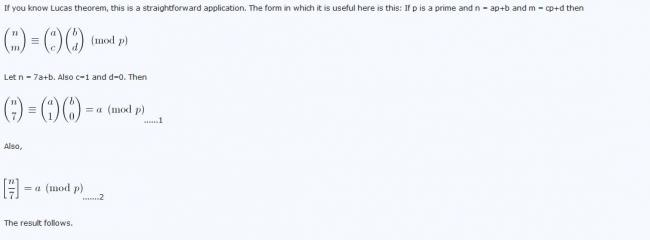# question

prove that for all integers, n > 7 C (n,7) - [n/7] is divisible by 7.
here [.] denotes the greatest integer less then or equal to the number in the bracket.
i tried it but all i could get was that for values of n in [7,13] the greatest integer function gave 1 and the combination part gave a number which left a number 1 when divided by 7.
similarly for n in [14,20] the remainder was 2. and the greatest integer function gave 2.
but i want a better approach.
••